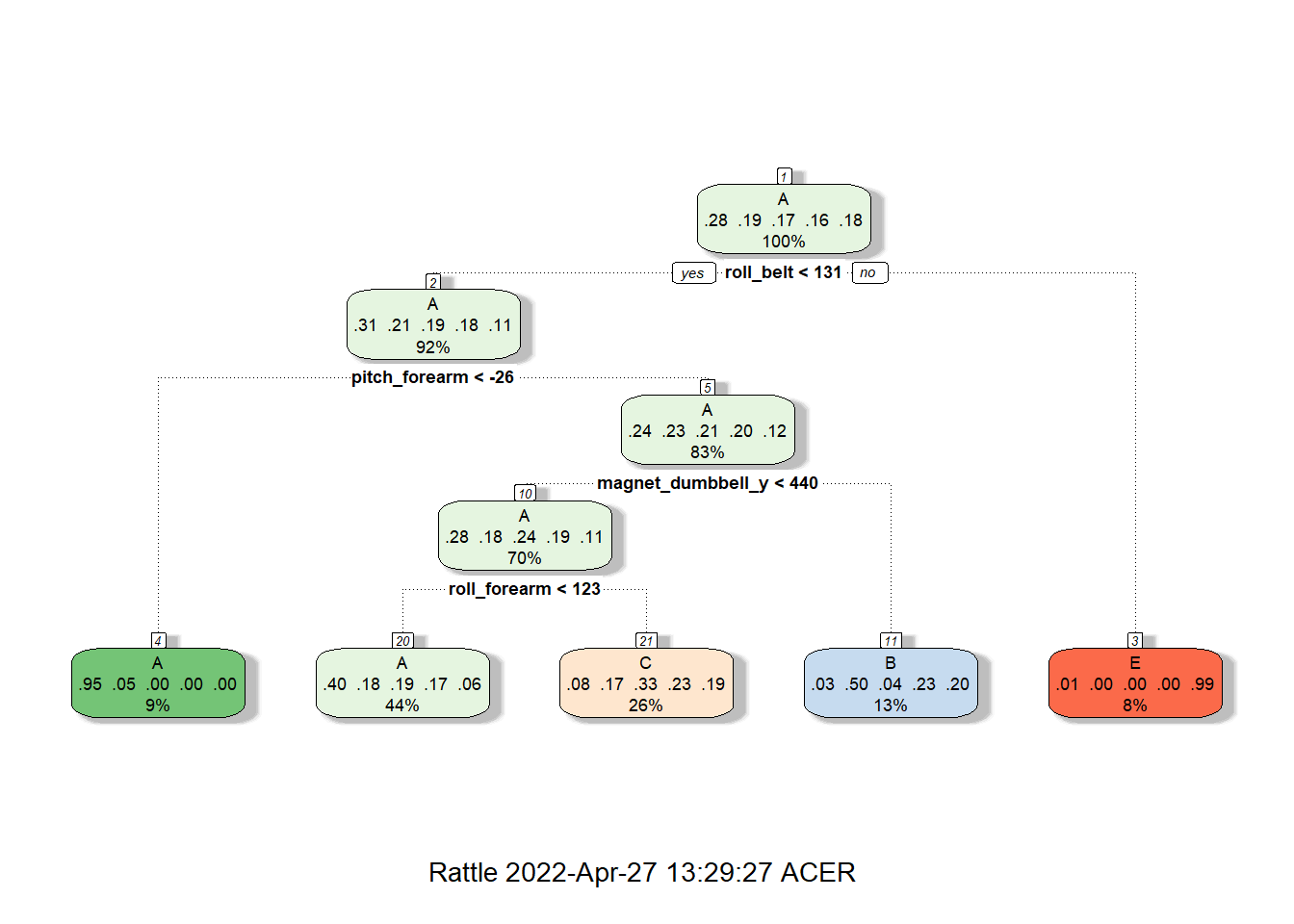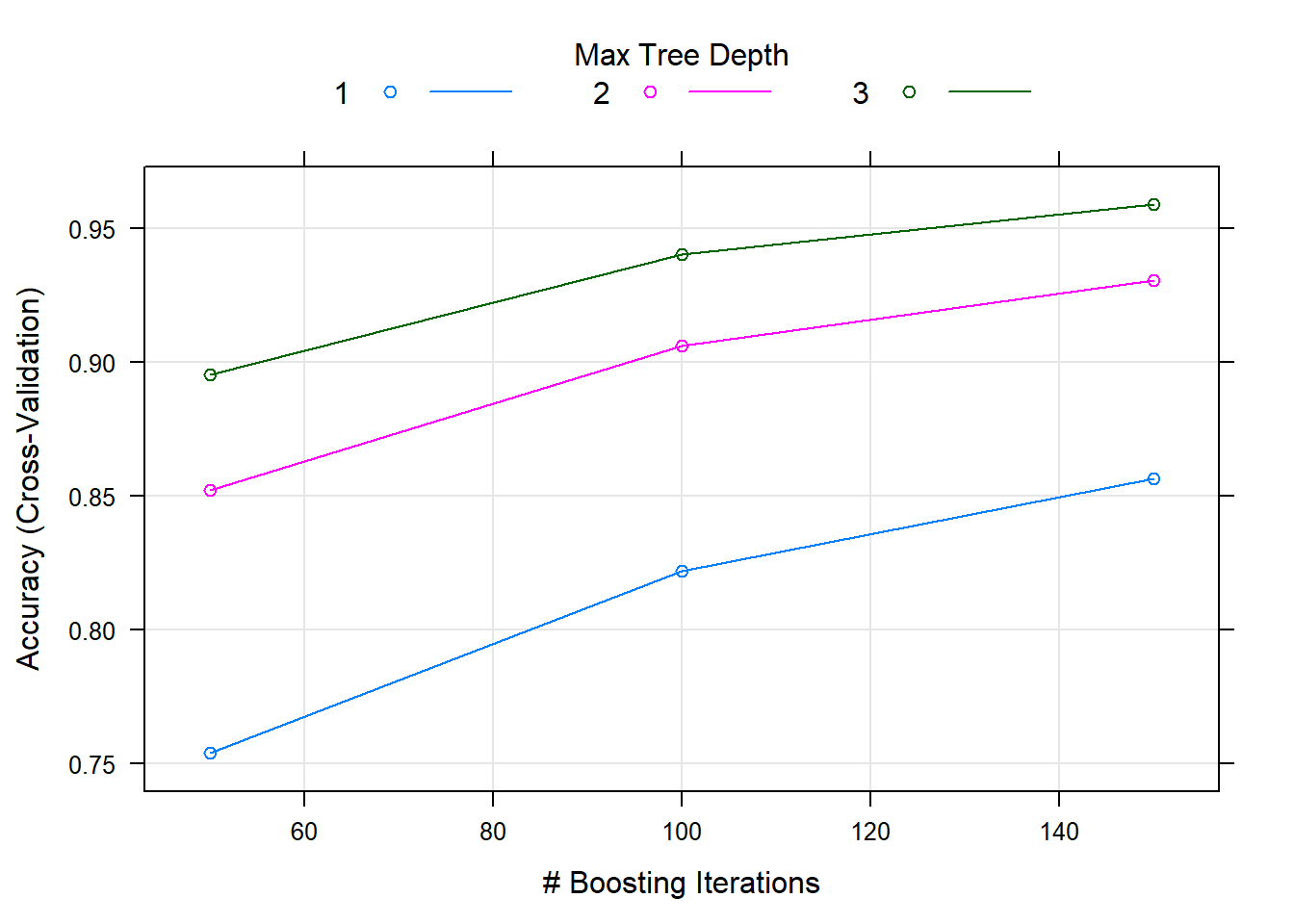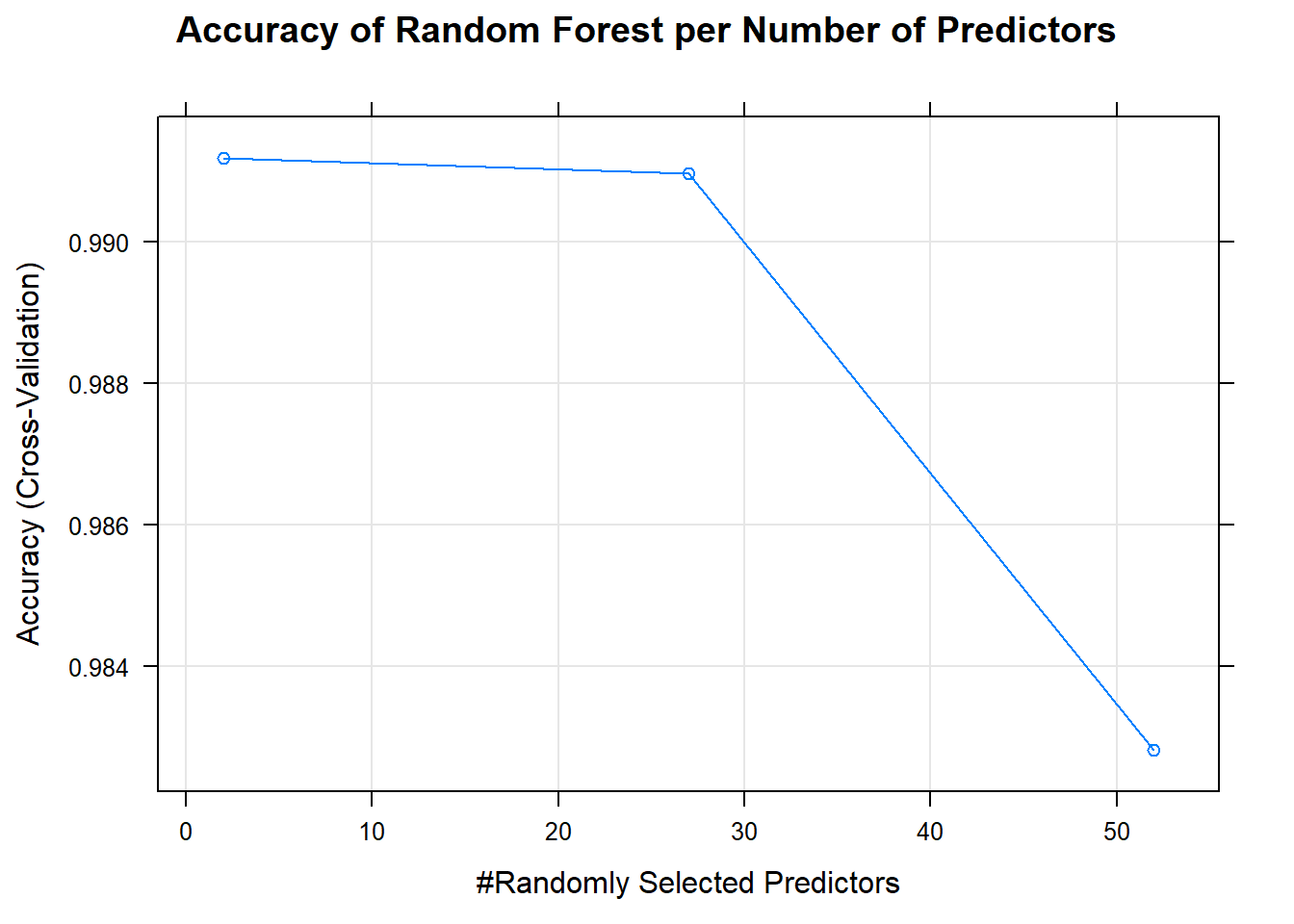## Overview

This project on practical machine learning works on a data set about the exercise movements of mobile users. In this project we will explore the data set, train machine learning models (using Classification Trees, Gradient Boost, and Random Forest), assess its respective accuracy in classifying these exercise movements using the validation set, and predict classifications made by the better performing model using the test set.

###Loading the required packages and the dataset for both training and testing

library(caret)
library(rattle)
library(randomForest)

testing <- read.csv("pml-testing.csv")

##Viewing the datasets to determine the structure of variables

##Results are hidden to save space
str(training);summary(training)
str(testing); summary(testing)
##training data has 19622 obs. of  160 variables
##testing data has 20 obs. of  160 variables
##It can also be observed that there were many columns that have NAs so it is time to clean the dataset.

##Cleaning the Dataset for both training and testing

##Removing unwanted columns; those with 90% NAs
RemoveNAtest <- which(colSums(is.na(training) |training == "")>0.9*dim(training))
RemoveNAtrain <- which(colSums(is.na(testing) |testing == "")>0.9*dim(testing))
Ctrain <- training[, -RemoveNAtrain]
Ctest <- testing[, -RemoveNAtest]

##Removing unwanted columns; those pertaining to user profile which is not relevant to prediction
Ctrain <- Ctrain[,-c(1:7)]
Ctest <- Ctest[,-c(1:7)]
##Both training and testing datasets now have 53 variables

##Data Splitting to remove out-of-sample errors

##Ctrain is split into 70% for training and 30% for validation
set.seed(7788)
inBuild <- createDataPartition(Ctrain$classe, p = 0.7, list = 0) Btrain <- Ctrain[inBuild,] Bval <- Ctrain[-inBuild,] ##Cross Validation ##Setting up the cross validation with 5-fold cross validation ##This improves model efficiency and limits overfitting set.seed(45) Ctrl <- trainControl(method = "cv", number = 5) ##Prediction Models This report considers three models for prediction which includes classification tree using “rpart”, random forest using “rf” and gradient boosting with “gbm” functions. ###Classfication Tree set.seed(11) modCT <- train(classe~., data = Btrain, method = "rpart", trControl = Ctrl) ##Create a nice plot fancyRpartPlot(modCT$finalModel)##Predict outcomes using the validation dataset
set.seed(11)
predCT <- predict(modCT, Bval)
##Check using Confusion Matrix
cmCT <- confusionMatrix(predCT, factor(Bval$classe)) aCT <- cmCT$overall['Accuracy']
print(round(aCT*100,2))
## Accuracy
##    50.21
##Low accuracy at 50.21% thus poor prediction of the outcome "classe"

set.seed(12)
modGBM <- train(classe~., data = Btrain, method = "gbm", trControl = Ctrl, verbose = 0)
##Create a nice plot
plot(modGBM)##Predict outcomes using the validation dataset
set.seed(12)
predGBM <- predict(modGBM, Bval)
##Check using Confusion Matrix
cmGBM <- confusionMatrix(predGBM, factor(Bval$classe)) aGBM <- cmGBM$overall['Accuracy']
print(round(aGBM*100,2))
## Accuracy
##     96.3
##We see a high accuracy at 96.3% but before we decide let us check the random forest

###Random Forest

set.seed(13)
modRF <- train(classe~., data = Btrain, method = "rf", trControl = Ctrl)
##Create a nice plot
plot(modRF, main = "Accuracy of Random Forest per Number of Predictors")##Predict outcomes using the validation dataset
set.seed(13)
predRF <- predict(modRF, Bval)
##Check using Confusion Matrix
cmRF <- confusionMatrix(predRF, factor(Bval$classe)) aRF <- cmRF$overall['Accuracy']
print(round(aRF*100,2))
## Accuracy
##    99.22
##Comparing the previous Accuracy we have computed, it looks like random
##forest has the highest accuracy at 99.22%. As such, we will be using
##random forest for prediction on the test dataset (Ctest).

##Prediction on Test dataset using Random Forest

Testpred <- predict(modRF, Ctest)
print(Testpred)
##   B A B A A E D B A A B C B A E E A B B B
## Levels: A B C D E

##Conclusion Three prediction models were considered to predict classe in the test dataset. These models include Classification Tree, Generalized Boosting Method and Random Forest. A 5-fold cross validation method was considered for efficiency purposes (faster processing) and to limit overfitting. Going over the specified models, the following accuracy were observed as 50.21% for Classification Tree, 96.3% for Generalized Boosting Method and 99.22% for Random Forest. This informs us that random forest has the lowest out-of-sample error at .0078 followed by Generalized Boosting Method at 0.037 and 0.4979 for Classification Tree. With the following findings, it is wise to decide on the use of Random Forest to predict classe in the test data set given its high accuracy and low out-of-sample error.